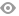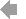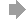﻿ Page 5 - REVISTA MATINF Nr. 5
Basic HTML VersionView Full Version
Page 5 - REVISTA MATINF Nr. 5
P. 5

``````˘
ARTICOLE SI NOTE DE MATEMATICA
,

A generalisation of a problem from Gazeta Matematic˘a

1
Leonard Giugiuc and Alexandru Daniel Pˆırvuceanu                 2

The following is the problem 27767 from Gazeta Matematic˘a, No. 11/2019:

27767. Let f : [0, 1] → R be a diﬀerentiable function with the property that

Z  1
f(x)dx = 0.
0
R 1
0
Prove that there exists c ∈ (0, 1) such that f (c) = −9   3  f(t)dt.
0
Marian Andronache and Dinu S , erb˘anescu, Bucharest

While we were solving this problem, we observed that a more general result holds. What is
interesting is that the oﬃcial solution published in  can be adapted to prove this more general
result. In this article, we aim to present this generalisation, followed by our solution:

Problem. Let a, b ∈ R, a < b. Consider a diﬀerentiable function f : [a, b] → R such that

Z  b
f(x)dx = 0.
a
Prove that for all x ∈ [a, b] there exists c x ∈ (a, b) such that

x
Z
0
2    f(t)dt = (x − a)(x − b)f (c x ).
a
Solution. If x = a or x = b, then there is nothing to prove, since the conclusion rewrites as
0 = 0.

For x ∈ (a, b), the conclusion is equivalent to proving that there exists c x ∈ (a, b) such that

Z  x
2    f(t)dt
0
a          = f (c x ).
(x − a)(x − b)

Z  y
Consider the function F : [a, b] → R, F(y) =      f(t)dt.
a
1
Professor, National ,,Traian” College, Drobeta-Turnu Severin, Romania, leonardgiugiuc@yahoo.com
2
Student, Faculty of Mathematics and Computer Science, University of Bucharest, Bucharest, Romania,
pirvuceanualexandrudaniel@gmail.com.

5``````1   2   3   4   5   6   7   8   9   10